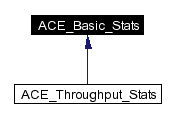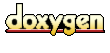Main Page   Namespace List   Class Hierarchy   Alphabetical List   Compound List   File List   Namespace Members   Compound Members   File Members   Related Pages

# ACE_Basic_Stats Class Reference

Collect basic stats about a series of samples. More...

`#include <Basic_Stats.h>`

Inheritance diagram for ACE_Basic_Stats:[legend]
List of all members.

## Public Methods

ACE_Basic_Stats (void)
Constructor.

ACE_UINT32 samples_count (void) const
The number of samples received so far.

void sample (ACE_UINT64 value)
Record one sample.

void accumulate (const ACE_Basic_Stats &rhs)
Update the values to reflect the stats in rhs.

void dump_results (const ACE_TCHAR *msg, ACE_UINT32 scale_factor) const
Dump all the samples.

## Private Attributes

ACE_UINT32 samples_count_
The number of samples.

ACE_UINT64 min_
The minimum value.

ACE_UINT32 min_at_
The number of the sample that had the minimum value.

ACE_UINT64 max_
The maximum value.

ACE_UINT32 max_at_
The number of the sample that had the maximum value.

ACE_UINT64 sum_
The sum of all the values.

ACE_UINT64 sum2_
The sum of the square of all the values.

## Detailed Description

Collect basic stats about a series of samples.

Compute the average and standard deviation (aka jitter) for an arbitrary number of samples, using constant space. Normally used for latency statistics.

## Constructor & Destructor Documentation

 ACE_INLINE ACE_Basic_Stats::ACE_Basic_Stats ( void )
 Constructor. The number of samples is pre-allocated, and cannot changes once the class is initialized.

## Member Function Documentation

 void ACE_Basic_Stats::accumulate ( const ACE_Basic_Stats & rhs )
 Update the values to reflect the stats in rhs.

 void ACE_Basic_Stats::dump_results ( const ACE_TCHAR * msg, ACE_UINT32 scale_factor ) const
 Dump all the samples. Prints out the results, using msg as a prefix for each message and scaling all the numbers by scale_factor. The latter is useful because high resolution timer samples are acquired in clock ticks, but often presented in microseconds.

 ACE_INLINE void ACE_Basic_Stats::sample ( ACE_UINT64 value )
 Record one sample.

 ACE_INLINE ACE_UINT32 ACE_Basic_Stats::samples_count ( void ) const
 The number of samples received so far.

## Member Data Documentation

 ACE_UINT64 ACE_Basic_Stats::max_` [private]`
 The maximum value.

 ACE_UINT32 ACE_Basic_Stats::max_at_` [private]`
 The number of the sample that had the maximum value.

 ACE_UINT64 ACE_Basic_Stats::min_` [private]`
 The minimum value.

 ACE_UINT32 ACE_Basic_Stats::min_at_` [private]`
 The number of the sample that had the minimum value.

 ACE_UINT32 ACE_Basic_Stats::samples_count_` [private]`
 The number of samples.

 ACE_UINT64 ACE_Basic_Stats::sum2_` [private]`
 The sum of the square of all the values.

 ACE_UINT64 ACE_Basic_Stats::sum_` [private]`
 The sum of all the values.

The documentation for this class was generated from the following files:
Generated on Fri Apr 2 16:47:18 2004 for ACE by1.2.18